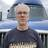Renumbering Subtable Records

I have a table named 'Apps Project' and a subtable named 'Trial Summary' that I want to be able to renumber with a button. This is only necessary if/when I've deleted records to throw off the numbering.

For example: I have T1, T2, T3, T4. I delete T1 and T3 and I'm left with T2 and T4. I want to be able to renumber those trials as T1 and T2 with the button formula.

I figure the best way of handling this is to capture the T#'s in an array, count the array and then create a new array that starts with 1 and ends with the count:

`let myArray := concat('Trial Summary'.'Apps Project'.'Trial Summary'.'T#');let myCnt := count(myArray);let newArray := concat(range(1, myCnt + 1));`

I'm not sure if this is the correct syntax, but more importantly, I don't know the correct syntax to then assign the values of newArray to the 'T#' column in the table.

I'm sure the solution is simple enough, but I'm not seeing it.

Any help would be greatly appreciated as I'm hoping to impliment this feature in multiple forms.I don't think you need to use an array. Is 'Trial Summary' orderd by the 'T#' or some other field?I realized it wouldn't make sense to order by 'T#' because T1, T10, etc would come before T2. So you could use something like this...

`let tNum := 1;`
`for r in (select 'Trial Summary') order by YourFieldName do`
`r.('T#' := "T" + tNum);`
`tNum := tNum + 1`
`end`

Sean,

I tried your code as is and it erased all 'T#' entries in the 'Trial Summary' table. I realized that it was probably because the code was not speaking to that specific iteration of the subtable. So, I did a quick "undo" and then modified what you provided with the code I already had to add entries to the subtable and it worked!

`let myID := this;let tNum := 1;for r in (select 'Trial Summary')['Apps Project' = myID].Id order by 'T#' do r.('T#' := tNum); tNum := tNum + 1`end

Greatly appreciate the help on this!William, Sorry about the scare and I'm glad you got it working. :)

Reply